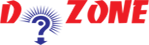﻿ How to make a simple calculator in Python?## Python Program to Make a Simple Calculator

Here is a simple example to create a simple calculator that can add, subtract, multiply or divide as per the input entered.

``````
Example
# Program make a simple calculator that can add, subtract, multiply and divide using functions

# This function adds two numbers
def add(x, y):
return x + y

# This function subtracts two numbers
def subtract(x, y):
return x - y

# This function multiplies two numbers
def multiply(x, y):
return x * y

# This function divides two numbers
def divide(x, y):
return x / y

print("Select operation.")
print("1.Addition")
print("2.Subtraction")
print("3.Multiplication")
print("4.Division")

# Take input from the user
choice = input("Enter choice(1/2/3/4):")

num1 = int(input("Enter first number: "))
num2 = int(input("Enter second number: "))

if choice == '1':
print(num1,"+",num2,"=", add(num1,num2))

elif choice == '2':
print(num1,"-",num2,"=", subtract(num1,num2))

elif choice == '3':
print(num1,"*",num2,"=", multiply(num1,num2))

elif choice == '4':
print(num1,"/",num2,"=", divide(num1,num2))
else:
print("Invalid input")
Output
Select operation.
1.Add
2.Subtract
3.Multiply
4.Divide
Enter choice(1/2/3/4): 4
Enter first number: 240
Enter second number: 8
240 / 8 = 30
```
```

So, to evaluate the operation, user have to choose the desired function like add(), subtract(), multiply() and divide() and as you see the result like you give the input the output appears on the screen.

PythonTraining, 258-FF , Katewa Nagar, New Sanganer Rd, Jaipur, 302019